# Two teas

A mixture weighing 10 kg was made from two types of tea. The price of tea was 160 CZK/kg and second tea 170 CZK/kg. The price of the mixture is 166 CZK/kg. How many kilograms of each type of tea had to be mixed?

Correct result:

a =  4 kg
b =  6 kg

#### Solution: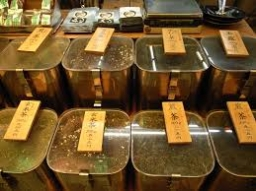We would be pleased if you find an error in the word problem, spelling mistakes, or inaccuracies and send it to us. Thank you!Tips to related online calculators
Do you have a system of equations and looking for calculator system of linear equations?

## Next similar math problems:

• Tea mixture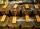Of the two sort of tea at a price of 180 CZK/kg and 240 CZK/kg we make a mixture 12 kg that should be prepared at a price of 200 CZK / kg. How many kilos of each sort of tea will we need to be mixed?
• Tea blendTea blends are maked from two kinds of tea. In standard tea mixture are two teas in the ratio 1:3 and 40 g costs 42 CZK. In the premium tea mixture are weighing two teas in the ratio 1:1 and 50 grams costs 60 CZK. How much cost 10 grams of more expensive
• MixingIf we mix 5 kg of goods of one kind and 3 kg second one, resulting mixture cost 16.50 EUR/kg. If these quantities are mixed in reverse - first three kilograms and 5 kilograms second cost of mixture is 18.50 EUR/kg. What is the price of one kg of goods of
• Coffee shop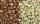To the coffee shop brought 2 types of coffee totally 50 kg. The first type was CZK 220 per kilogram, coffee second type 300 CZK per 1 kg. For all the coffee trader earned CZK 12,000. How many kilograms of coffee of first type and how many kilograms of cof
• SweetsWe want to prepare 5 kg of sweets for 150 CZK. We will mix cheaper candy: 1 kg for 120 CZK and more expensive candy: 1 kg per 240 CZK. How much of this two types of candy is necessary to prepare this mixture?
• Coffee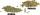Coffee merchant has coffee robusta and arabica species. 1 kg Robusta worth 450 CZK, Arabica 1 kg is 300 CZK more expensive. Calculate how many kilograms of Robusta and Arabica will need to produce 30 kg of the mixture so that the mixture cost is 490 CZK p
• Bonbons 2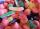Kilo sweets will cost 260 CZK. The first type has a price per 320 kg, the second type 240 CZK per kg. How many kilos of both kinds of sweets need to prepare a 100 kg mixture ?
• Candies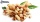In the confectionery, the price for 1 kg of pistachio candies cost CZK 360, and the price for 1 kg of hazelnut candies was CZK 280. Mixing these two types of sweets created a box of chocolates. How many grams of pistachios and how many grams of hazelnut c
• Mixture of nutsThe mixture of nuts should be prepared from almonds, peanuts and cashew nuts ratio 1: 2: 3 (respectively). The price of almonds is 150 CZK/kg, the price of peanuts is 140 CZK/kg and the price of cashew nuts is 180 CZK/kg. The price of the mixture is deter
• CandiesThe price for 1 kg of more expensive candies is 125 CZK. The price for 1 kg of cheaper candy is 100 CZK. We mix two different mixtures of candy. A) The first mixture contains 2 kg more expensive and 0.5 kg cheaper candy. Calculate the price for 1 kg of thThe fruit salad is prepared from oranges and bananas. One kilogram of oranges costs 30 CZK and one kilogram of bananas costs 18 CZK. How many kilograms of bananas need to be added to half a kilogram of oranges to produce a fruit salad worth CZK 21 per 1 kThe price for 1kg of more expensive candy is 125 CZK. The price for 1kg of cheaper candy is 100 CZK. We make two different blends of candy. And now. The second blend contains 2kg of more expensive candy and several kg of cheaper candy. The price per 1 kgTwo types of alcohol one 63% and second 75% give 20 liters of 69% alcohol. How many liters of each type are in the mixture?In what ratio must two sorts of sugar, costing #390 and #315 per kg respectively, be mixed in order to produce a mixture worth #369 per kg?If a person weighs 80 kg, how many kilograms of cork must take swimming belt to use it to float on water? The density of the human body is 1050kg/m3 and cork 300kg/m3. (Instructions: Let the human body and cork on a mixture that has a density of 1000kg/m3From 55% and 80% alcohol we have to produce 0.2 kg of 60% alcohol. How many of them do we use in solution?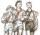Scouts purchased for camp cans two types total cost 1460 CZK. First cans were for CZK 32 and second for 25 CZK. How many cans purchased each type?